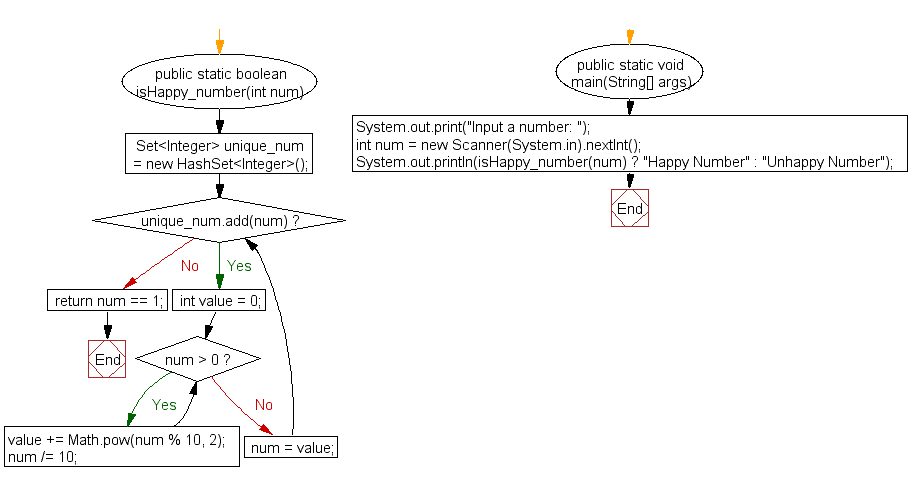﻿ Java exercises: Check whether a given number is a happy number or unhappy number - w3resource# Java Exercises: Check whether a given number is a happy number or unhappy number

## Java Numbers: Exercise-10 with Solution

Write a Java program to check whether a given number is a happy number or unhappy number.

Happy number: Starting with any positive integer, replace the number by the sum of the squares of its digits, and repeat the process until the number equals 1, or it loops endlessly in a cycle which does not include 1.
An unhappy number is a number that is not happy.
The first few unhappy numbers are 2, 3, 4, 5, 6, 8, 9, 11, 12, 14, 15, 16, 17, 18, 20.

Pictorial Presentation:Sample Solution:

Java Code:

``````import java.util.HashSet;
import java.util.Scanner;
import java.util.Set;

public class Example10 {

public static boolean isHappy_number(int num)
{
Set<Integer> unique_num = new HashSet<Integer>();

{
int value = 0;
while (num > 0)
{
value += Math.pow(num % 10, 2);
num /= 10;
}
num = value;
}

return num == 1;
}

public static void main(String[] args)
{
System.out.print("Input a number: ");
int num = new Scanner(System.in).nextInt();
System.out.println(isHappy_number(num) ? "Happy Number" : "Unhappy Number");
}
}
```
```

Sample Output:

```Input a number: 5
Unhappy Number
```

Flowchart:Java Code Editor:

What is the difficulty level of this exercise?

﻿

## Java: Tips of the Day

Array vs ArrayLists:

The main difference between these two is that an Array is of fixed size so once you have created an Array you cannot change it but the ArrayList is not of fixed size. You can create instances of ArrayLists without specifying its size. So if you create such instances of an ArrayList without specifying its size Java will create an instance of an ArrayList of default size.

Once an ArrayList is full it re-sizes itself. In fact, an ArrayList is internally supported by an array. So when an ArrayList is resized it will slow down its performance a bit as the contents of the old Array must be copied to a new Array.

At the same time, it's compulsory to specify the size of an Array directly or indirectly while creating it. And also Arrays can store both primitives and objects while ArrayLists only can store objects.

Ref: https://bit.ly/3o8L2KH